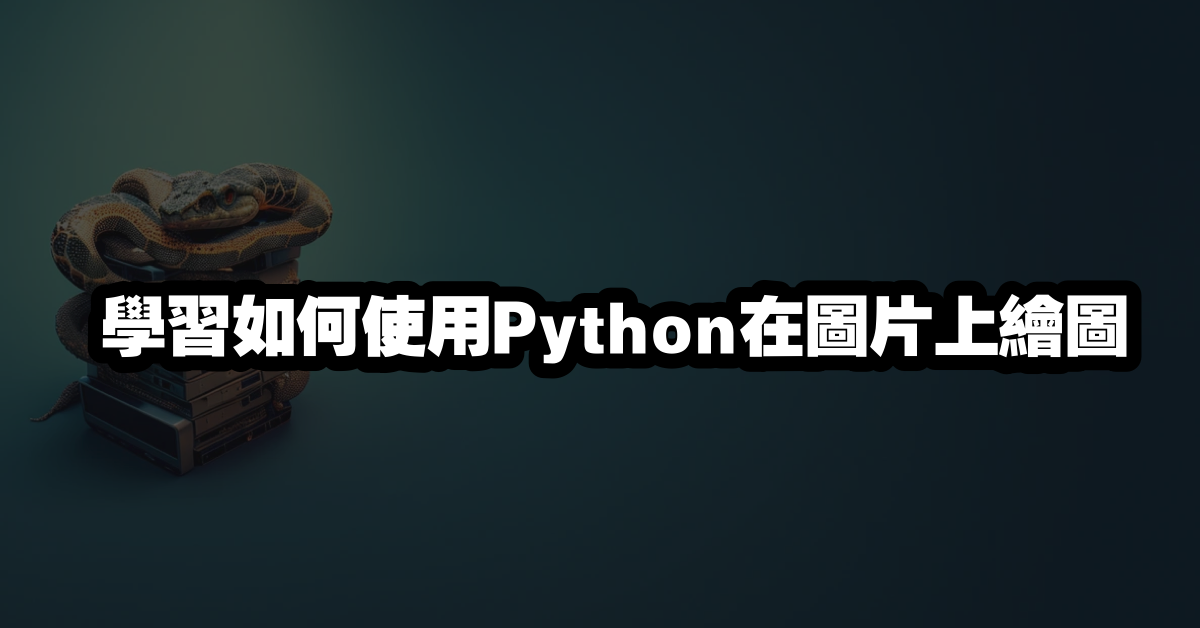# 學習如何使用Python在圖片上繪圖# 如何使用 Python 在圖片上繪圖

Python 是一種流行的程式語言，它可以用於開發各種應用程式，包括圖片上的繪圖。本文將介紹如何使用 Python 來在圖片上繪圖，以及一些有用的繪圖技巧。

## 使用 Python 繪圖的基本步驟

pip install Pillow


from PIL import Image


img = Image.open('image.jpg')


## 繪製簡單的圖形

from PIL import Image, ImageDraw

# 開啟一張圖片
img = Image.open('image.jpg')

# 建立畫布
draw = ImageDraw.Draw(img)

# 繪製一條直線
draw.line((0, 0) + img.size, fill=(255, 255, 255))

# 儲存圖片
img.save('image_line.jpg')


## 繪製多邊形

from PIL import Image, ImageDraw

# 開啟一張圖片
img = Image.open('image.jpg')

# 建立畫布
draw = ImageDraw.Draw(img)

# 繪製一個三角形
draw.polygon([(250, 250), (300, 300), (200, 300)], fill=(255, 255, 255))

# 儲存圖片
img.save('image_polygon.jpg')


## 繪製文字

from PIL import Image, ImageDraw, ImageFont

# 開啟一張圖片
img = Image.open('image.jpg')

# 建立畫布
draw = ImageDraw.Draw(img)

# 設定字型
font = ImageFont.truetype('arial.ttf', size=20)

# 繪製文字
draw.text((250, 250), 'Hello World!', font=font, fill=(255, 255, 255))

# 儲存圖片
img.save('image_text.jpg')


## 繪製圖案

from PIL import Image, ImageDraw, ImageFont

# 開啟一張圖片
img = Image.open('image.jpg')

# 建立畫布
draw = ImageDraw.Draw(img)

# 開啟一張圖案
pattern = Image.open('pattern.jpg')

# 繪製圖案
draw.bitmap((250, 250), pattern)

# 儲存圖片
img.save('image_pattern.jpg')